# Use Scaled Doubles to Avoid Precision Loss

This example shows how you can avoid precision loss by overriding the data types in your model with scaled doubles.

### Open the Model

Open the `ex_scaled_double` model.

```ex_scaled_double ```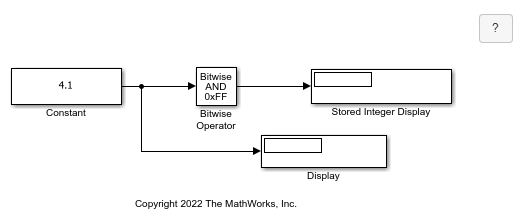In the `ex_scaled_double` model, the Constant block Output data type is `fixdt(1,8,4)`. The Bitwise Operator block uses the `AND` operator and the bit mask `0xFF` to pass the input value to the output. Because the Treat mask as parameter is set to Stored Integer, the block outputs the stored integer value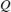of its input. The encoding scheme is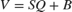, where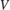is the real-world value andis the stored integer value.

### Collect Ranges in the Fixed-Point Tool

1. From the Simulink® Apps tab, select Fixed-Point Tool.

2. Select the Range Collection workflow.

3. In the Fixed-Point Tool, select Collect Ranges > Use current settings. Click Collect Ranges.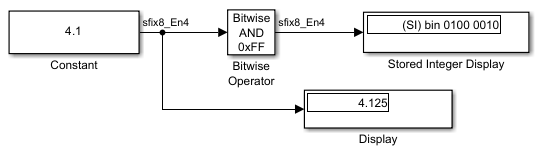The Display block displays `4.125` as the output value of the Constant block. The Stored Integer Display block displays `(SI) bin 0100 0010`, which is the binary equivalent of the stored integer value. Precision loss occurs because the output data type, `fixdt(1,8,4)`, cannot represent the output value `4.1` exactly.

### Override Data Types with Scaled Doubles

Override data types in the model with scaled doubles.

1. In the Fixed-Point Tool, select New > Range Collection to start a new workflow.

2. Select Collect Ranges > Scaled double precision. Click Collect Ranges.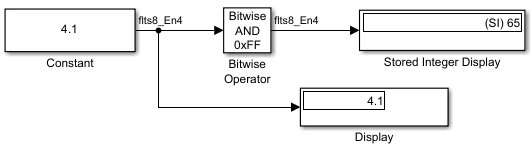The Display block correctly displays `4.1` as the output value of the Constant block. The Stored Integer Display block displays `(SI) 65`, which is the binary equivalent of the stored integer value. Because the model uses scaled doubles to override the data type `fixdt(1,8,4)`, the compiled output data type changes to `flts8_En4`, which is the scaled double equivalent of `fixdt(1,8,4)`. No precision loss occurs because the scaled doubles use a double to hold the stored value and retain information about the specified data type and scaling. Note that you cannot use a data type override setting of Double precision or Single precision on this model because the Bitwise Operator block does not support floating-point data types.